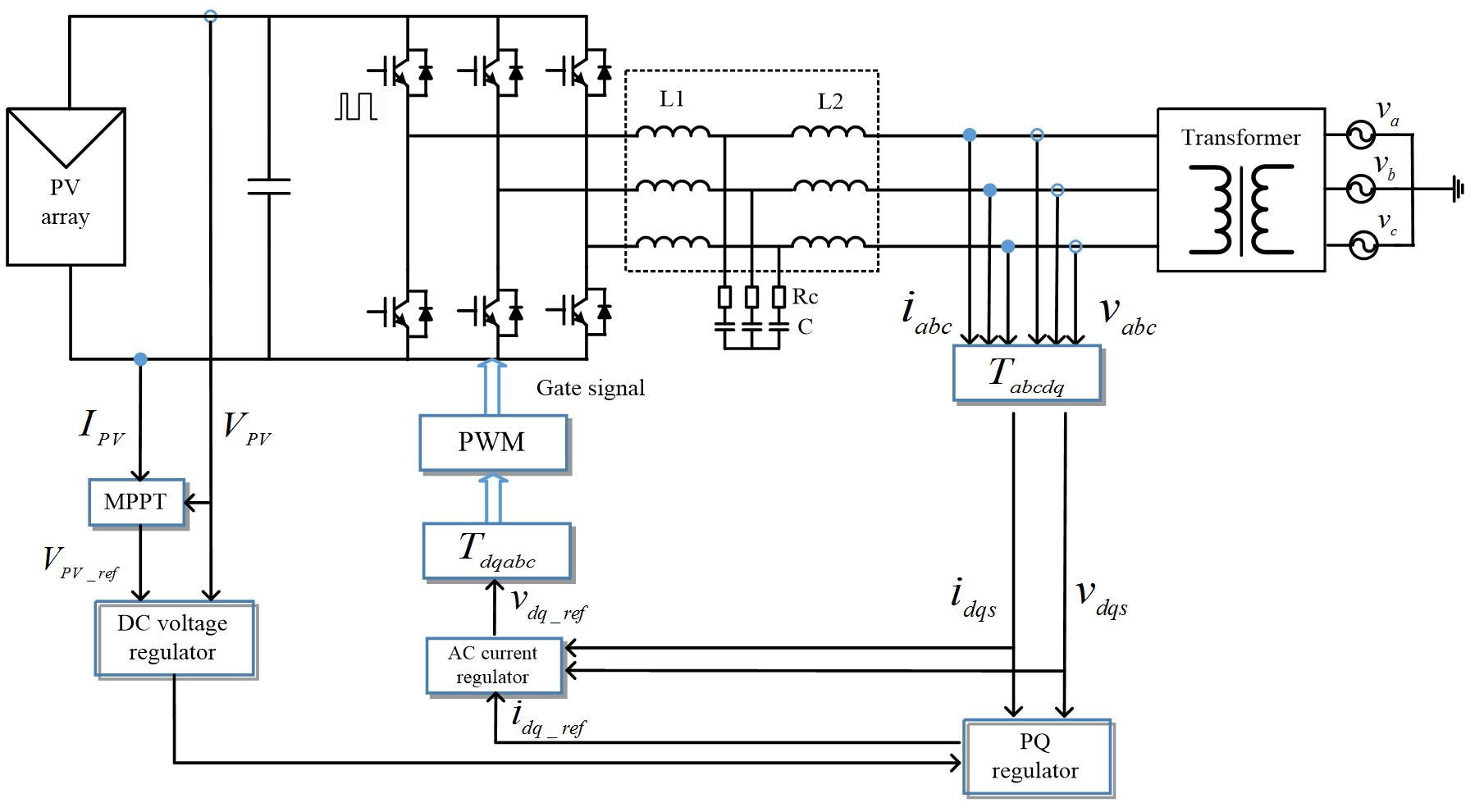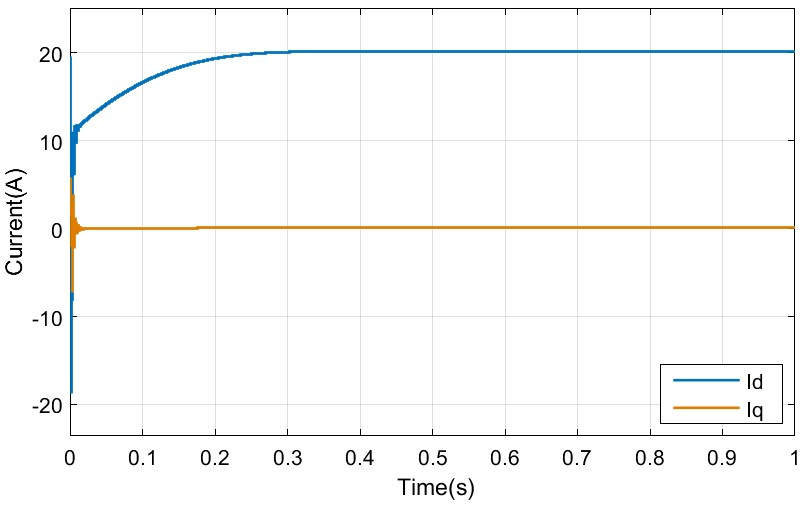Contact Us
LIBRARY

# AC Impedance Derivation of Utility Scale PV FarmFig. 1. Topology of utility PV
Since negative incremental resistance caused by the constant power behavior of power converters may incur stability problems for distributed systems, the stability of photovoltaic (PV) integration to distributed systems has attracted increasing attention. In contrast to real-time simulation or the character root method, both of which require full modeling of all components in distributed systems, a generalized Nyquist stability criterion (GNC) for stability analysis of three-phase ac power systems uses only measured dq-frame impedances. Since the control of the PV inverter has not only inner d-axis and q-axis current loops, but also has a dc voltage loop, it is more accurate for assessing integration stability using the dq-frame impedances, versus positive and negative sequence impedances. To assess the stability of utility PV farm integration, a switching model and an average model for a 250 kW inverter module in a utility PV farm are built in MATLAB. The PV output impedance on the dq-frame is derived and calculated based on linearization of the average model, including dynamics of power stage, current and voltage controllers and PLL. The derived impedance matches the simulation results obtained from the linearization function of MATLAB. In addition, hardware experiments of scaled-down PV inverter impedance measurements validate the impedance in the dq-frame derived from linearization of the average model. PV output impedances derived in a combination of grid impedances are used in the application of GNC. Time domain simulations based on the average model prove that the GNC method is able to accurately predict system stability. The oscillation frequency of the time domain matches with the frequency-domain analysis obtained by using characteristic loci of minor-loop gain in the GNC. The reactive power control PV farm may significantly influence system stability if the PV inverter is under Q control mode, i.e., Q = f(V).Fig. 2. (a) Time domain simulation results of PV currentFig. 2. (b) Q = f(V)
INDUSTRY PARTNERS